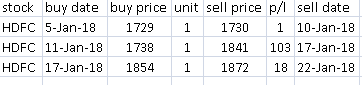# How to calculate buy and sell average

i was back testing a strategy, i don’t know how to calculate average buy and sell price, please write me correct method of calculating average buy and sell price from below screen shot. thanks1 Like

@Anshu_Tiwari : Purchase price average is 1773.66 and sell price average is 1814.33. ADD all 3 of your purchase divide by 3 . same way do it for your sale.

@sabkaview thanks for reply, one more query -
as you wrote to divide by “3” , what is 3 here? total no. of quantity or total no. of transaction?

total number of quantity should be divided by total value of purchase or sell as the case may be to arrive at average price.

1 Like

Weighted average based on quantity

Summation (P,Q) / Summation (Q)